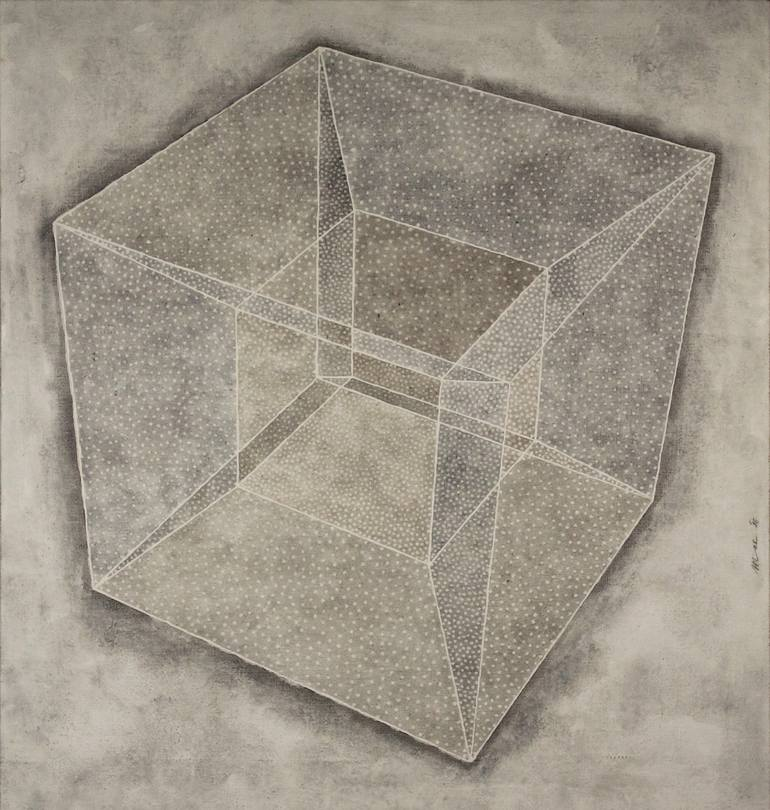# Eigenvalues of subgraphs of the cube

Hamming balls, subgraphs of the hypercube, maximise the graph’s largest eigenvalue exactly when the dimension of the cube is large enough.

European Journal of Combinatorics 70, 125 (2018)

B. Bollobás, J. Lee, S. LetzterWe consider the problem of maximising the largest eigenvalue of subgraphs of the hypercube Q d of a given order. We believe that in most cases, Hamming balls are maximisers, and our results support this belief. We show that the Hamming balls of radius o ( d ) have largest eigenvalue that is within 1 + o ( 1 ) of the maximum value. We also prove that Hamming balls with fixed radius maximise the largest eigenvalue exactly, rather than asymptotically, when d is sufficiently large. Our proofs rely on the method of compressions..